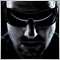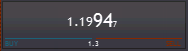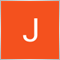Delayed drawing and chart updating with MT5/MQL5 - page 41233

The Class definition is CBidAsk : public CWndBmd : public CWnd : public CVObject : public CObject : public CStruct

Embedded objects:

The m_Canvas object is CCanvasExt : public CCanvas : public CObject : public CStruct

The m_symbol object is CSymbolInfoExt : pubic CSymbol : public CObject : public CStruct

Example of output/result:Source of OnRedraw()

It starts with a time-measurement which ensures, that the update will not be executed more often than the human eye can recognize (20fps). Afterwards some light shading is applied with another function which uses a "bit" of math too ;)

The class does not execute a ChartRedraw() - it just sets a flag that the chart needs to be redrawn.

protected:
//+------------------------------------------------------------------+
//|  Redraw content                                                  |
//+------------------------------------------------------------------+
virtual bool            OnRedraw(void)
{
//--- Implement a timer execution which results in an update not more
//    often than every n msecs, as specified in constructor

IMPLEMENT_TIMER_EXECUTION_REALTIME_NOTONMOUSEFOCUS(return false)

#ifdef __MQL4__
#endif
//--- Displayed main price
double displayprice=0;
switch(m_showprices)
{
case BIDASK_SHOW_LAST   : displayprice=m_symbol.Last(); break;
case BIDASK_SHOW_BID    : displayprice=m_symbol.Bid(); break;
}

//--- Bid & ask price
{
m_price1=m_symbol.Bid();
}
else
{
m_price2=m_symbol.Bid();
}

//--- Vars
int x1=0;
int y1=0;
int x2=m_rect.Width()-1;
int y2=m_rect.Height()-1;
string price;
uint argbBrd=COLOR_TO_RGBA(m_colorborder);
uint argbB  = m_colorbg;

bool swappedpos = BIDASK_SWAPPED;

//--- Check positions
uint argbL  = swappedpos ? m_color2 : m_color1;
uint argbR  = swappedpos ? m_color1 : m_color2;
uint argbLB = swappedpos ? COLOR_TO_RGBA(m_colorclick2) : COLOR_TO_RGBA(m_colorclick1);
uint argbRB = swappedpos ? COLOR_TO_RGBA(m_colorclick1) : COLOR_TO_RGBA(m_colorclick2);
string textL = swappedpos ? m_textBuy : m_textSell;
string textR = swappedpos ? m_textSell : m_textBuy;

COLOR_RGBA color1=m_color1;
COLOR_RGBA color2=m_color2;

//--- Show both?
m_ybase=y2;
m_xmid=x2/2;
int x2leftprice = showboth ? m_xmid : x2;
int x1rightprice = showboth ? m_xmid : x1;

//--- Erase
m_Canvas.Erase();
//--- Draw rectangular area
if (m_colorborder!=clrNONE)
else

//--- Lower area prepare
if (m_spacebelow>0)
{
m_ybase=m_ybase-m_spacebelow;
m_Canvas.LineHorizontal(x1+3,x2-4,m_ybase,argbBrd);
}

{

price=_DoubleToString(ABS(m_price2-m_price1)+m_symbol.PointsToPrice(m_symbol.CommissionSpreadPts()),m_digits==0 ? 1 : m_digits) + " (abs)";
else

price+=" *";

}

//--- Show red/green line or one
//    At least lower area or spread must be shown
if (m_showspread || m_spacebelow>0)
{
if (m_clickactive || showboth)
{
m_Canvas.LineHorizontal(x1+3,m_xmid-2,m_ybase,argbLB);
m_Canvas.LineHorizontal(m_xmid+3,x2-4,m_ybase,argbRB);
}
else
{
m_Canvas.LineHorizontal(x1+3,x2-4,m_ybase,argbBrd);
}
}

//--- Middle line
if (showboth)
m_Canvas.LineVertical(m_xmid,y1+3,m_ybase-3,argbBrd);

//--- Button pressed
int xshiftL=0, yshiftL=0;
int xshiftR=0, yshiftR=0;

if (m_mousedown==1 && VO_IS_ACTIVE)
{
int xright = showboth ? m_xmid : x2;
uint edge = showboth ? ROUNDRECT_EDGE_LT : ROUNDRECT_EDGE_LT|ROUNDRECT_EDGE_RT;
xshiftL=1;
yshiftL=1;
if (!showboth)
{
xshiftR=1;
yshiftR=1;
displayprice=m_price1;
}
textR=NULL;
if (_ColorBrightness(argbLB)>0.5)
{
color1=0;
color2=0;
}
}
else if (m_mousedown==2 && VO_IS_ACTIVE)
{
int xleft = showboth ? m_xmid : x1;
uint edge = showboth ? ROUNDRECT_EDGE_RT : ROUNDRECT_EDGE_LT|ROUNDRECT_EDGE_RT;
xshiftR=1;
yshiftR=1;
if (!showboth)
{
xshiftL=1;
yshiftL=1;
displayprice=m_price2;
}
textL=NULL;
if (_ColorBrightness(argbRB)>0.5)
{
color1=0;
color2=0;
}
}

if (m_showlabels)//!m_showboth && m_clickactive)
{
}

//--- Prices (bid/ask already swapped in Price())
//    For the case we want to see the ask only, we need to re-swap
double price1=m_price1;
double price2=m_price2;

SWAP(price1,price2,double);

//--- Calculate font size
m_fontsize=m_Canvas.PixelsToFontSize(int((m_ybase-y1)*0.48));
int fontsize= showboth ? m_fontsize : (int)(double(m_fontsize*1.2));
int fontsizebig = (int)(double(fontsize*1.5));
int fontsizesmall = (int)(double(fontsize*0.6));

m_Canvas.FontSet(m_fontname,fontsize);

if (showboth || !swappedpos)
{
//--- Price left
price=showboth ? _DoubleToString(price1,m_digits) : _DoubleToString(displayprice,m_digits);
int len=StringLen(price);
int bignumbers=MIN(len-m_smallnumbersright,m_bignumbersright);
price="size;"+(string)fontsize+";text;"+STR_SUBSTR(price,0,len-bignumbers-m_smallnumbersright)+";size;"+(string)(fontsizebig)+";text;"+STR_SUBSTR(price,len-bignumbers-m_smallnumbersright,bignumbers)+";size;"+(string)fontsizesmall+";text;"+STR_SUBSTR(price,len-m_smallnumbersright,-1)+";";
m_Canvas.TextOutRectFmt(x1+xshiftL+2,y1+yshiftL+6,x2leftprice-2+xshiftL,m_ybase+yshiftL,price,color1,ALIGN_CENTER,ALIGN_TOP);
}
//--- Exit if not both prices are shown
if (showboth || swappedpos)
{
// Price right   2
price=showboth ? _DoubleToString(price2,m_digits) : _DoubleToString(displayprice,m_digits);
int len=StringLen(price);
int bignumbers=MIN(len-m_smallnumbersright,m_bignumbersright);
price="size;"+(string)fontsize+";text;"+STR_SUBSTR(price,0,len-bignumbers-m_smallnumbersright)+";size;"+(string)(fontsizebig)+";text;"+STR_SUBSTR(price,len-bignumbers-m_smallnumbersright,bignumbers)+";size;"+(string)fontsizesmall+";text;"+STR_SUBSTR(price,len-m_smallnumbersright,-1)+";";
m_Canvas.TextOutRectFmt(x1rightprice+xshiftR,y1+yshiftR+6,x2-2+xshiftR,m_ybase+yshiftR,price,color2,ALIGN_CENTER,ALIGN_TOP);
}
return true;
}39054

If you say it.1233

?39054

Doerk Hilger:

?

I am trying to help. What can I do with your previous post ? nothing...

I also tried your code with MathMod() and didn't get an answer ?1233

You asked for some sample source code and I posted it?! If you take a look at the class definition, you see that its impossible to post all the code which is executed during a drawing process cause it´s nested too deep. I cannot post something that you can compile just like that.

About MathMod ... this is just an example. You can increase the loop count and use anything else to make your own tests.39054

Doerk Hilger:

You asked for some sample source code and I posted it?! If you take a look at the class definition, you see that its impossible to post all the code which is executed during a drawing process cause it´s nested too deep. I cannot post something that you can compile just like that.

About MathMod ... this is just an example. You can increase the loop count and use anything else to make your own tests.

If you can't even post a working example, I can't help.

I really hope you will find solution(s).1233

Alain Verleyen:

If you can't even post a working example, I can't help.

I really hope you will find solution(s).

I will somehow as always. Thank you.81

There is a solution for this.

First you must disable auto scroll. Then you will get a tiny grey triangle at the bottom of the chart. Move this triangle to the bar you want to use as the "lock".

Now when you zoom, it will stay in place, on the selected bar. When you change timeframe, it is reset though.

You can also use "home" and "end" buttons to navigate to first and last bar of the chart.

Hope this helps!